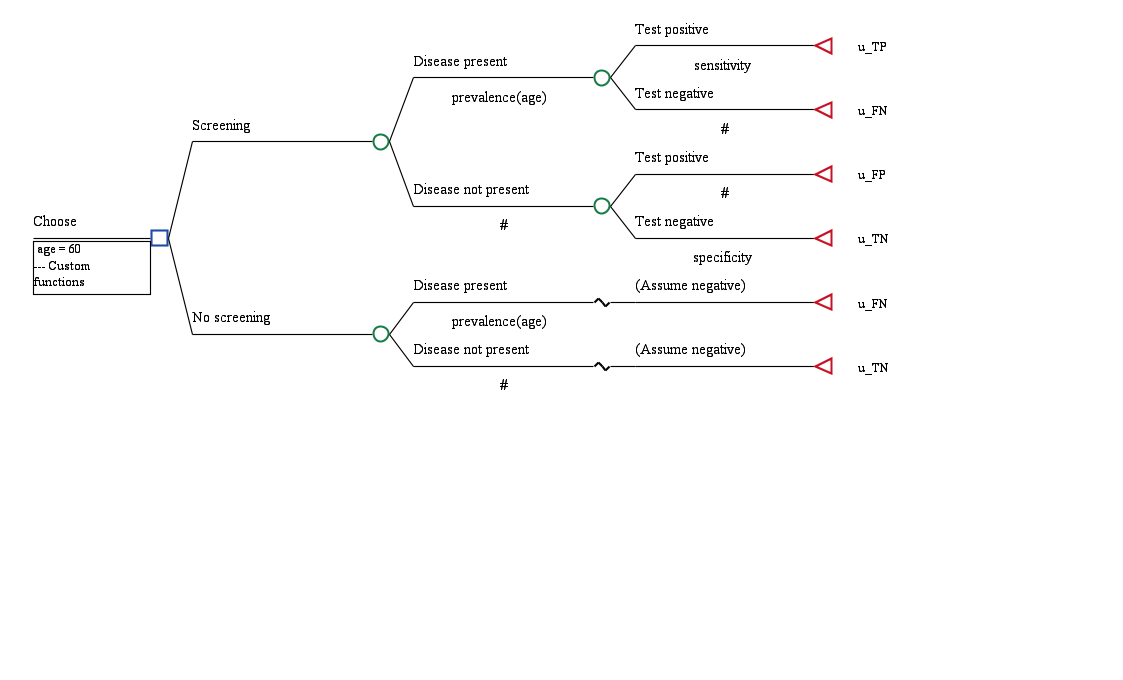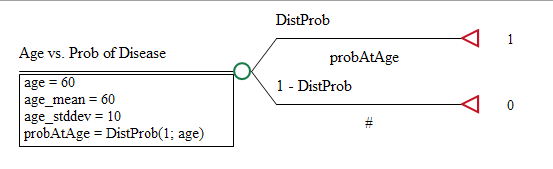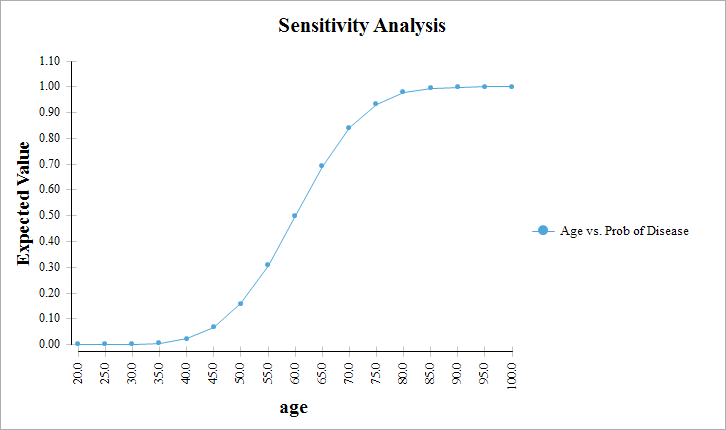# Probability density function for distribution

Hello,

I know I can use DistProb to get the cumulative distribution function for a distribution, and can use DistValue to get the inverse cumulative distribution function (the quantile function).

My question is, is there a similar function for the probability density or probability mass?

These are generally not too challenging as formulae to implement by hand but it would make my model a lot cleaner if I could just use DistDensity or DistMass or DistFunction with an existing distribution!

Thanks

• It is true that we do not have a function that returns a point on the probability density function (PDF) or probability mass function (PMF). We had not anticipated that anyone would need that. Can you describe how you would use this value in a model?

We do have a DistTransProb function that returns the probability of an even occurring in any time range from a time-to-event distribution.

I created an article and example model to try to demonstrate the three distribution functions DistProb, DistValue, and DistTransProb.

https://treeage.zendesk.com/hc/en-us/articles/115002041513-Distribution-Functions-DistProb-DistValue-DistTransProb

• Hi Andrew,

In my particular example I was determining the prevalence of a condition being screened for according to the age of the patient. The age distributions for people with and without the condition are approximately normal, so I was using Bayes' rule as follows:

p(Condition|Age) = p(Age|Condition) p(Condition)/p(Age) = p(Age|Condition) p(Condition) / (p(Age|Condition) p(Condition) + p(Age|¬Condition) p(¬Condition))

Where p(Age|Condition) and p(Age|¬Condition) are both normal distribution density functions.

• That's an interesting application via Bayes' rule.

I think that DistTransProb is the function you need. If you refer to the article I referenced earlier, you will see that the DistTransProb function calculates the probability for any time period for a distribution that measures time-to-event. Note that the further into the future, the probability will move closer and closer to 1.

I believe you have two normal distributions for time-to-event. The question remains as to what period you need. If you are running a Markov model, it will be the current age with a period equal to the cycle length.

Once you have the probabilities from DistTransProb, you can feed those into your Bayes' rule formulas.

• Thanks.

So if I understand correctly, DistTransProb is calculating a conditional failure probability, i.e., 1 - S(t+dt|t). Dividing this by dt and multiplying by S(t) gives an approximation to f(t), but this is not necessary if I have DistTransProb on the top and bottom of the fraction as these factors cancel out. I think it is therefore correct that I can use DistTransProb in this case and choose a suitably short period (short enough for the approximation to be valid but not too short to run into numerical issues). I have already hand-coded the probability density function so it is not a current issue for me, but I will bear in mind this option next time!

• I think you need DistTransProb regardless of the cycle length. If the cycle length is short enough, DistTransProb will return something very close to 0.

Do you have a Markov model? If so, are you using these functions to determine the probability of an event in a Markov cycle (for now ignoring the Bayes element). If so, then DistTransProb is part of your answer.

I believe that the PDF does not actually yield probabilities until you start evaluating area under the curve. Then it gets a little more complicated because there may not be much room left in the CDF toward later periods, so the probability starts to move toward 1.

• It's not a Markov model, but a simple decision tree (shown below), where we are screening for a risk factor/disease which is associated with age. The person being tested has a point age (60) rather than a distribution of ages, and by Bayes' theorem we calculate the probability of the disease being present as I described above.

f(x) can be approximated with DistTransProb as follows:

DistTransProb(x; \delta x) = 1 - S(x + \delta x | x) = 1 - S(x + \delta x)/S(x)

S(x) DistTransProb(x; \delta x) = S(x) - S(x + \delta x) = F(x + \delta x) - F(x)

S(x) DistTransProb(x; \delta x) / (\delta x) = (F(x + \delta x) - F(x)) / (\delta x)

lim_(x -> 0) LHS = f(x)• After looking at your model, I think we may have made this more complicated than necessary. I also may have led you astray with the DistTransProb function.

If you can create a single normal distribution that represents the distribution of ages at which the disease is first present, then the DistProb function will return the probability that the disease is present at any given age.

Consider this simple model.The model includes a normal distribution with mean age_mean and standard deviation age_stddev. If run at the base level, the model will return 0.50 because exactly 50% of the population will have the disease at the mean age for the distribution.

If I run sensitivity analysis on age, you can see that as the age increases, the probability of the disease also increases according to the PDF function via DistProb.If this would be useful, I can send you this model via a separate channel since I cannot attach a model to this thread.

It is also possible that I am misinterpreting what you need.